# 数字滤波器设计——MATLAB仿真篇1

1. IIR滤波器（无限冲激响应滤波器）
下面是MATLAB给出的一些IIR滤波器的调用方式：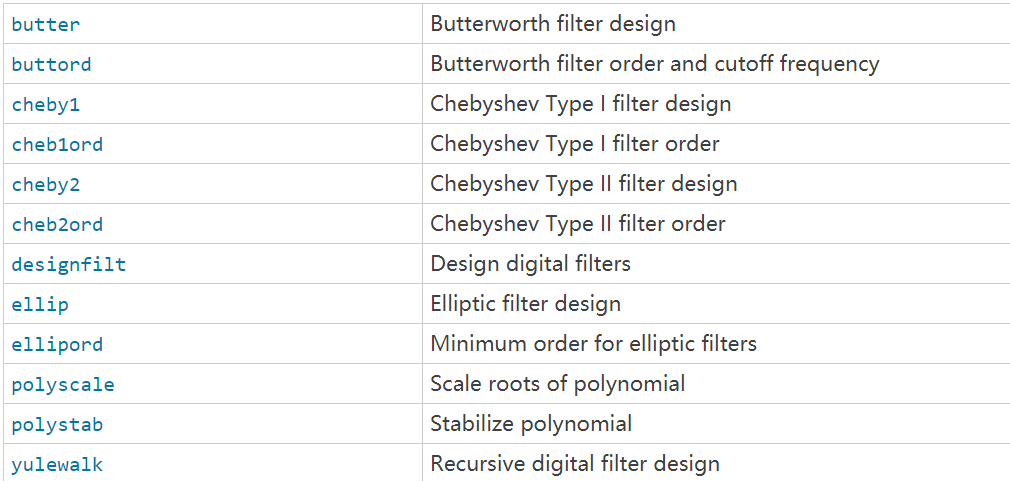%% 巴特沃斯低通滤波
% create by Grass
%% 滤波器参数
fs=20000;%采样频率
N=201;%采样点数
t=(0:N-1)/fs;%采样时间
%% 产生xn和hn
f1=600;
f2=2000;
x=sin(2*pi*f1*t)+sin(2*pi*f2*t);
figure;
plot(t,x);
title('叠加两个频率的输入信号'),xlabel('时间/s'),ylabel('幅度');
%巴特沃斯低通滤波器设计
Wp=f1/(fs/2); %通带截止频率
Ws=f2/(fs/2); %阻带截止频率
[n,Wn]=buttord(Wp,Ws,3,60);
[B,A]=butter(n,Wn);%调用巴特沃斯函数
%% 观察巴特沃斯函数形状
figure;
freqz(B,A);
%% 进行卷积
y=filter(B,A,x);
figure;
plot(t,y);
title('滤波之后的函数'),xlabel('时间/s'),ylabel('幅度');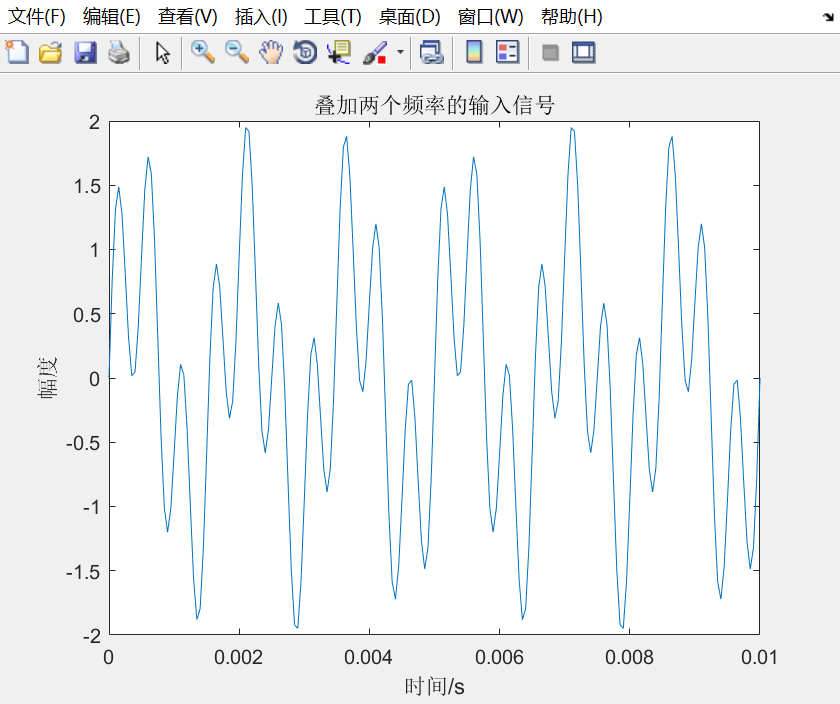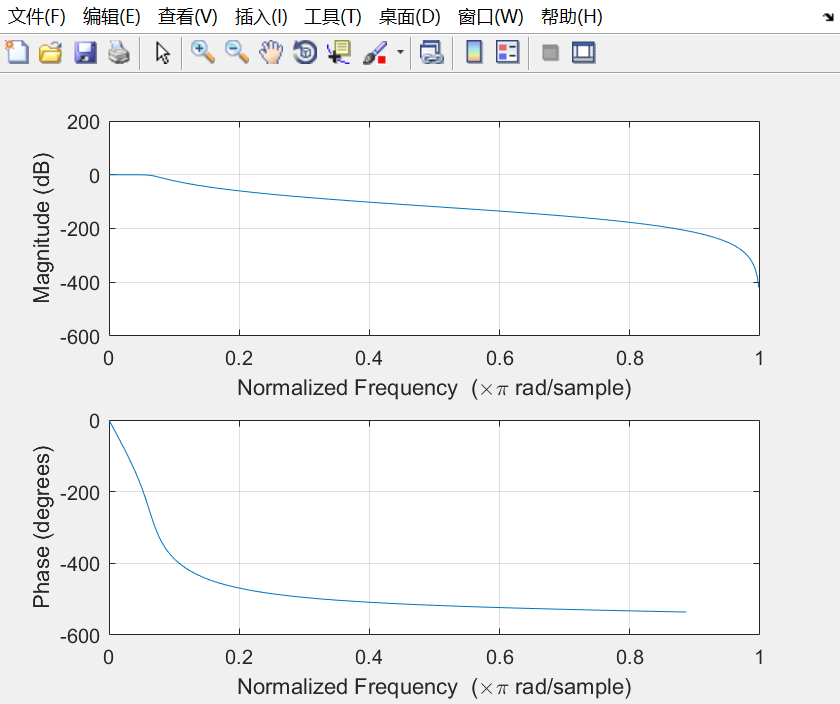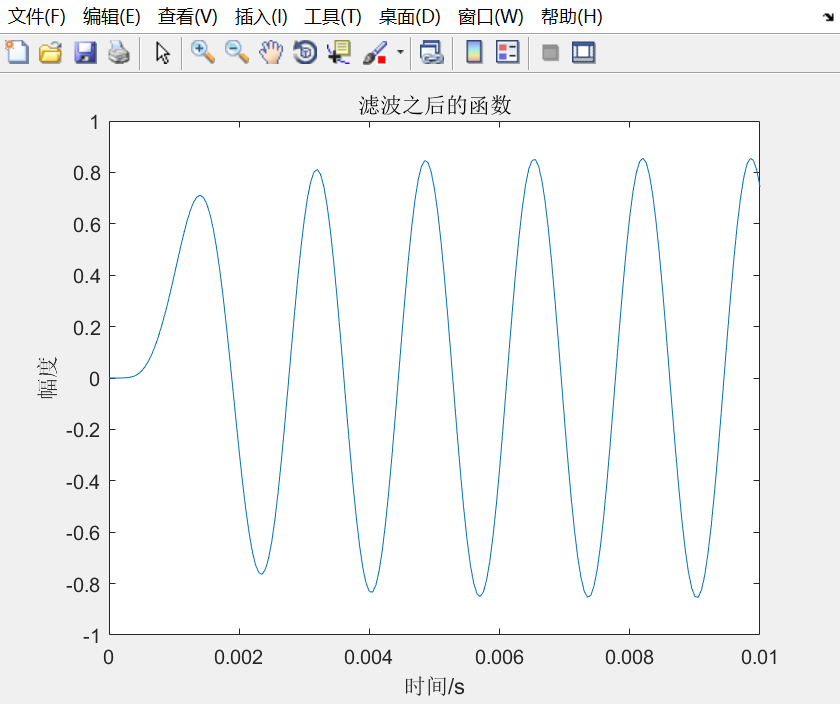1. FIR滤波器（有限冲击响应滤波器）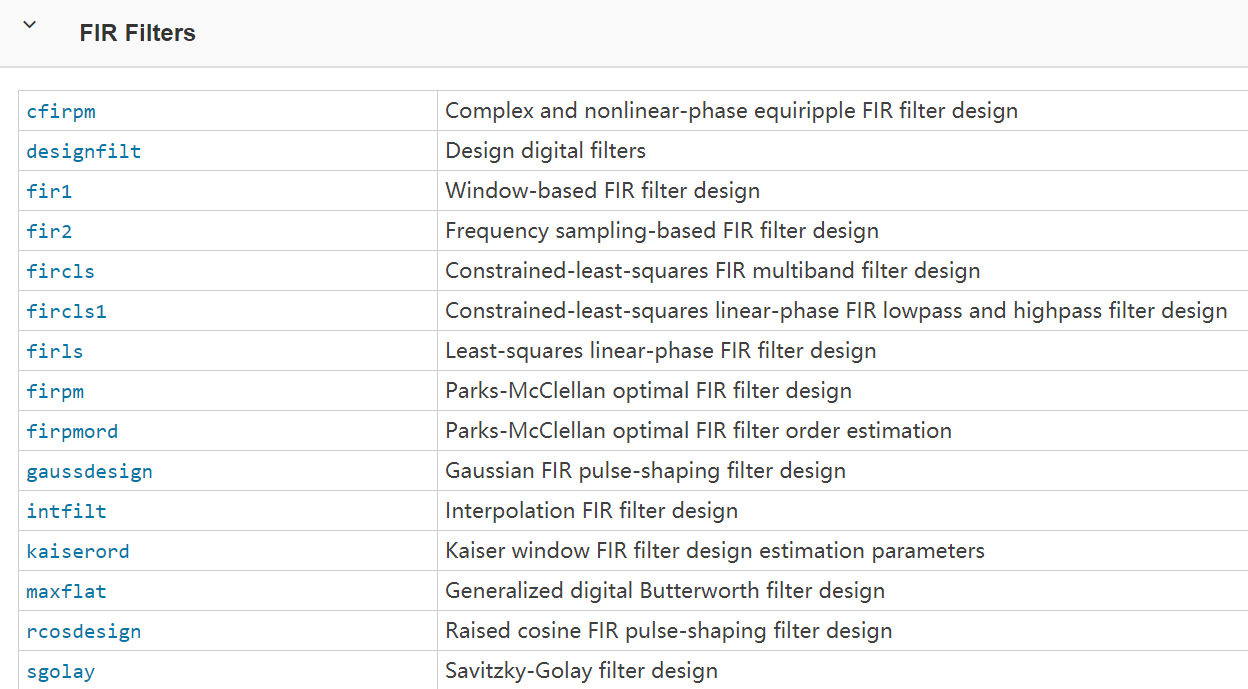b = fir2(n,f,m)

%% FIR滤波器设计
% create by Grass
%% 滤波器参数
fs=20000;%采样频率
N=201;%采样点数
t=(0:N-1)/fs;%采样时间
%% 产生xn和hn
f1=600;
f2=2000;
x=sin(2*pi*f1*t)+sin(2*pi*f2*t);
figure;
plot(t,x);
title('叠加两个频率的输入信号'),xlabel('时间/s'),ylabel('幅度');
%FIR滤波器设计
f=[0,f1/fs,f1/fs,1];
m=[1,1,0,0];
b1=fir2(25,f,m);
[h1,w]=freqz(b1,1);
figure;
plot(w/pi,abs(h1))
xlabel('\omega / \pi');
%% 进行卷积
y=filter(4*b1,1,x);%增益补偿
figure;
plot(t,y);
title('滤波之后的函数'),xlabel('时间/s'),ylabel('幅度');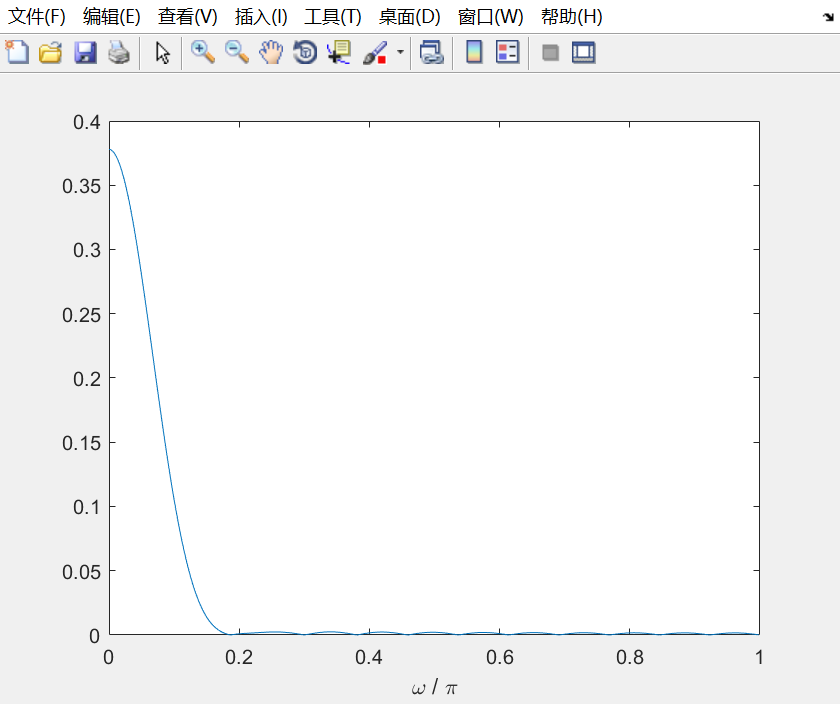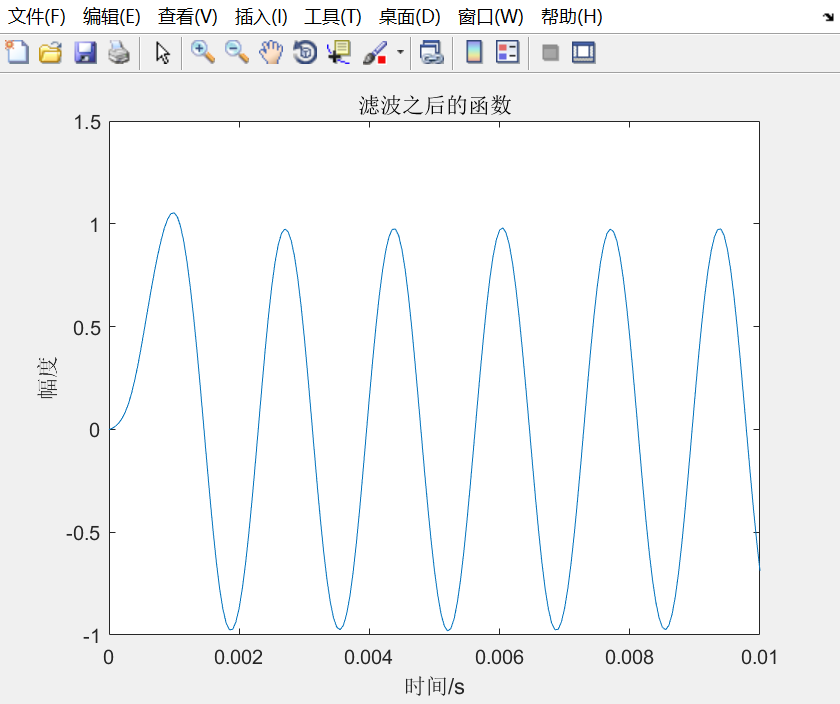08-0715603-1641
03-1719
03-10
01-059451
11-273万+
01-084万+
11-281594
03-18367
01-185402
01-204437
07-261万+
11-176473
12-041万+点击重新获取扫码支付余额充值